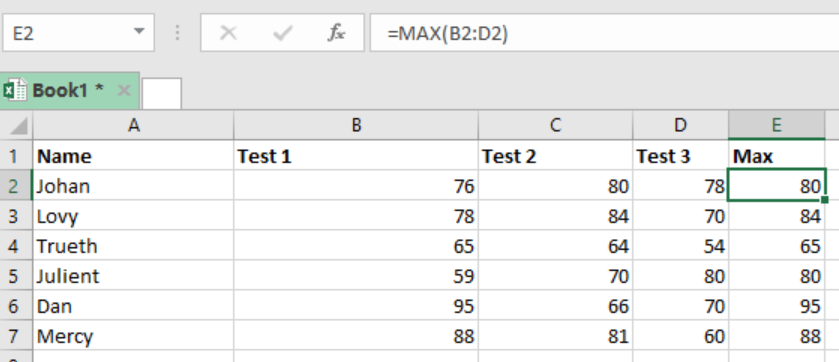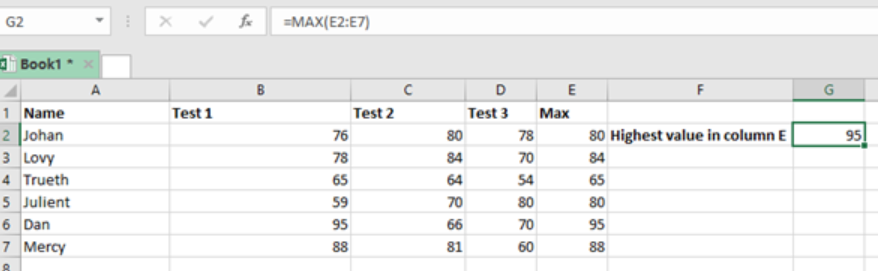Get instant live expert help with Excel or Google Sheets“My Excelchat expert helped me in less than 20 minutes, saving me what would have been 5 hours of work!”

#### Post your problem and you’ll get Expert help in seconds.

Your message must be at least 40 characters
Our professional Expert are available now. Your privacy is guaranteed.

# Maximum value

We can use the MAX function to get the maximum value in a given set of numbers. This post provides a clear way on how to go about it.Figure 1: Finding maximum value in a row

## Syntax of the formula

`=MAX (range)`

Where;

•         Range refers to the range from which we want to find the maximum value

## How the function works

The MAX function in excel is among the easiest and perhaps the most commonly used. It is often used to get the highest value in a given data. Usually, this formula can only work with numeric values, otherwise it will return an error. We can use it to get the highest value either in a row or column.

• When working with a row, we shall specify the range row-wise, and if it column, then it will be column-wise.
• We shall apply the formula ow-wise if we want to get the highest value an individual got.
• We use the column wise formula if we want to know who got the highest value.

## Explanation of figure 1 above

In figure 1 above, we want to find the maximum score each student got in the three tests. To do this, proceed as follows;

• In cell E2, put the formula =MAX (B2:D2). This shows that we are checking the maximum value in row 2.
• Press Enter to get the highest value.
• Copy down the formula to get the highest values for the other rows

## ExampleFigure 2: Finding maximum value in a column

In this example, we want to establish the highest value column-wise. This basically helps us find the highest score among the test. To do this, we simply write the formula in the cell where we want to get our result. In our case, it is cell G2. We then press Enter to get the result.

## Instant Connection to an Expert through our Excelchat Service

Most of the time, the problem you will need to solve will be more complex than a simple application of a formula or function. If you want to save hours of research and frustration, try our live Excelchat service! Our Excel Experts are available 24/7 to answer any Excel question you may have. We guarantee a connection within 30 seconds and a customized solution within 20 minutes.

### Did this post not answer your question? Get a solution from connecting with the expert.Another blog reader asked this question today on Excelchat:## Subscribe to Excelchat.coAnother blog reader asked this question today on Excelchat: